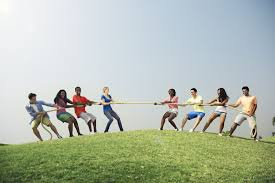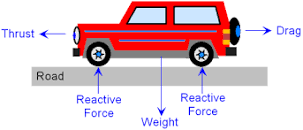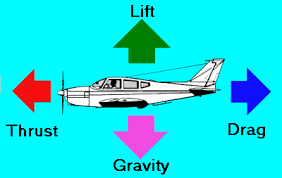## 2020-06-09

### Effects of Forces

1. In physics, a force is any interaction that, when unopposed, will change the motion of an object. A force can cause an object with mass to change its velocity (which includes to begin moving from a state of rest), i.e., to accelerate, to change its moving direction, shape etc. A force has both magnitude and direction, making it a vector quantity.(1)

2. The usual symbol of force is F and its SI unit is Newton (N).

3. As mentioned, a force will be able to change the shape, size, state of motion, direction of motion, acceleration of an object or body.

4. In our daily lives, the effects of forces can be seen everywhere, from driving a car, pushing a trolley, riding a bicycle, swimming, jumping, flying, gliding, rolling and even when stopping a movement.

5. A force is deemed to be balanced when a forces cancel out each other resulting in net zero force. A good example is during a tug-of-war competition when both sides of the opponents have the same pulling force resulting in no net movement.  When this happens the force is said to be balanced.6. When a balanced force is acting on an object, the object will remain stationary or moving at a constant velocity. E.g car moving at a constant velocity and plane flying with a constant velocity. There is no acceleration.

7. Hence, when a system is in unbalanced force, the object will experience acceleration.

8. Bear in mind that there can be more than two forces acting on a body at a certain time. For example below are the forces acting on a car and plane.

9. The movement of the object depends on the direction of the force / forces that have higher magnitude,(1) Wikipedia

## 2020-05-25

### Application for the principles of momentum conservation

1.       Billiard / Pool game

Billiards game uses a ball to hit other balls into holes. The more momentum the cue ball has the greater the momentum force exerted on the target ball / balls.

2.       Water hose

Water that jets out from a firemen hose exerts high backward force, hence firemen needs to hold fast the hose to prevent it from moving.

3.       Boat

When rowing a boat, the backward force exerted by the rower will result in the same forward force being produced.

4.       Jet engine

The hot gas and vapour ejected at the exhaust of a jet engine will produce an upward and forward force, equal to the force exerted by the hot gas.

5.       Rocket

A mixture of oxygen and hydrogen are burned up in the combustion chamber. This creates a very high pressure and force which is expelled at the bottom of the rocket. As a result, an upward force of similar magnitude is produced.

## 2020-05-15

### Linear momentum collision problem calculations 2

Questions

1.     A toy truck with a mass of 3 kg was moving at 3 ms-1 and hit with another toy truck of 1 kg and was moving with a velocity of 1 ms-1, in the opposite direction. After collision, both trucks moved together in the same direction, calculate the common velocity of the two objects after collision.

m1u1 + m2u2 =  (m1+ m2)v

(3)(3) + (1)(-1) = (3+1)v

9 – 1 = 4v

v = 2 ms-1

2.     A hunter shot a 100g bullet from a 1.5kg gun. If the bullet travelled 200 ms-1 after being triggered, what is the backward jerk of the gun. (Think final backward velocity of the gun).

Total initial momentum = Total final momentum = 0

m1u1 + m2u2 = 0

1.5(v) + 0.1(200) = 0 ß make sure to convert 100g to kg

1.5v +20 = 0

v = - 13.3 ms-1   ß Value is negative because gun moved in opposite direction

3.     A trolley of mass 2 kg was in a stationary condition, before a 5 g sticky plasticine was thrown into it with a velocity of 500 ms-1. After hitting the trolley, the plasticine sticks into it. Calculate the final velocity of both the trolley and plasticine?

Use the classic formula of momentum conservation

m1u1 + m2u2 = m1v1 + m2v2

0.005 (500) + 2 (0) = (0.005+ 2)v

2.5 = 2.005v

v = 1.245 ms-1